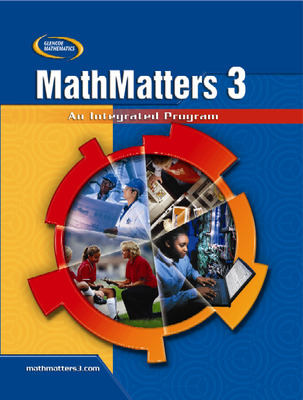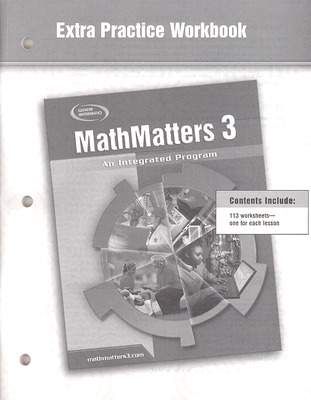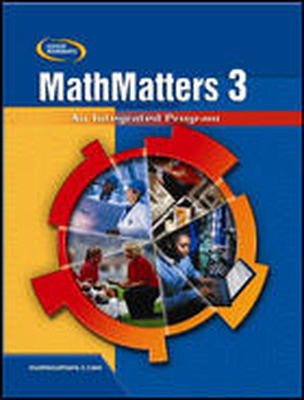# MathMatters 3: An Integrated Program

The MathMatters series covers Algebra 1, Geometry, and introductory Algebra 2 content as well as measurement, probability, and statistics.Features:

• Engage students with Chapter Investigations and hands-on activities.
• Increase student interest in mathematics with over 1,000 consumer, career, technical, and professional applications.
• Familiarize your students with the types of question and formats they will face on local, state, and national tests with two pages of Standardized Test Practice per chapter.
• Increase students’ access for additional math help with Glencoe’s extensive online resources, including Self-Check Quizzes, Extra Examples, and Standardized Tests aligned to their MathMatters text.

Contents:

MathMatters 3

• Chapter 1 Essential Mathematics
• Chapter 2 Essential Algebra and Statistics
• Chapter 3 Geometry and Reasoning
• Chapter 4 Triangles, Quadrilaterals and Other Polygons
• Chapter 5 Measurement
• Chapter 6 Linear Systems of Equations
• Chapter 7 Similar Triangles
• Chapter 8 Transformations
• Chapter 9 Probability and Statistics
• Chapter 10 Right Angles and Circles
• Chapter 11 Polynomials
• Chapter 13 Advanced Functions and Relations
• Chapter 14 Trigonometry

## Program Components

•MathMatters 3: An Integrated Program, Extra Practice Workbook
•MathMatters 3: An Integrated Program, StudentWorks DVD
Sort by: